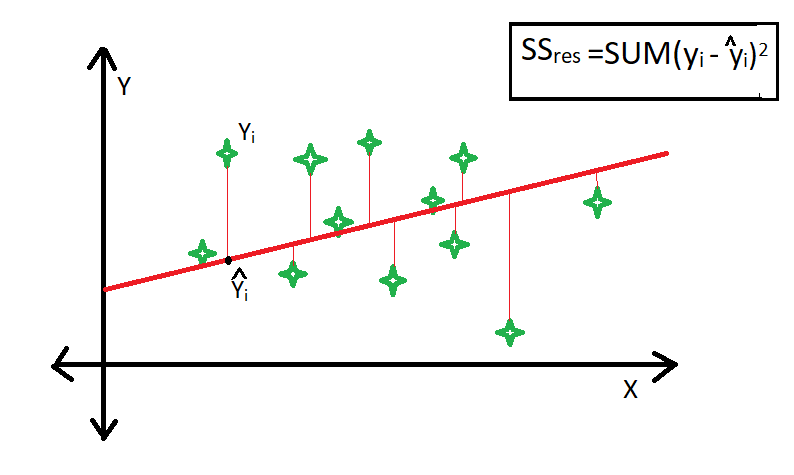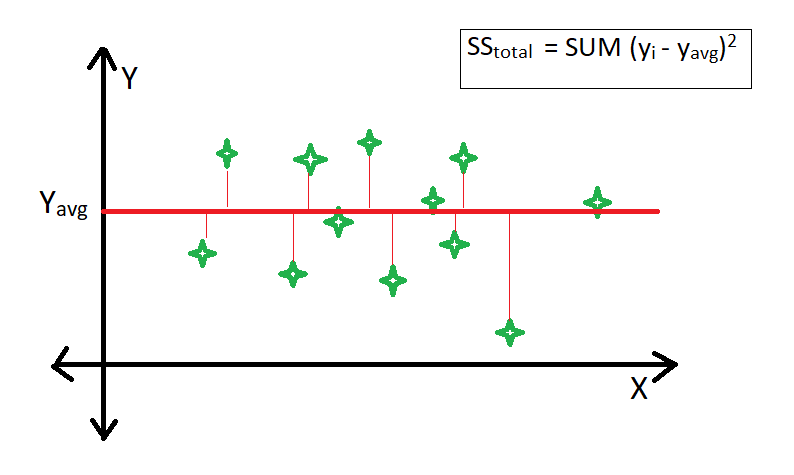Skip to content
Related Articles
R-squared Regression Analysis in R Programming
• Last Updated : 28 Jul, 2020

For the prediction of one variable’s value(dependent variable) through other variables (independent variables) some models are used that are called regression models. For further calculating the accuracy of this prediction another mathematical tool is used, which is R-squared Regression Analysis or the coefficient of determination. The value of R-squared is between 0 and 1. And if the coefficient of determination is 1 (or 100%) means that prediction of the dependent variable has been perfect and accurate.

R-square is a comparison of the residual sum of squares (SSres) with the total sum of squares(SStot). The residual sum of squares is calculated by the summation of squares of perpendicular distance between data points and the best-fitted line.The total sum of squares is calculated by the summation of squares of perpendicular distance between data points and the average line.#### Formula for R-squared Regression Analysis

The formula for R-squared Regression Analysis is given as follows,where,: experimental values of the dependent variable: the average/mean: the fitted value

#### Find the Coefficient of Determination(R) in R

It is very easy to find out the Coefficient of Determination(R) in the R language. The steps to follow are:

• Make a data frame in R.
• Calculate the linear regression model and save it in a new variable.
• The so calculated new variable’s summary has a coefficient of determination or R-squared parameter that needs to be extracted.
 # Creating a data frame of exam marksexam <- data.frame(name = c("ravi", "shaily",                             "arsh", "monu"),                   math = c(87, 98, 67, 90),                   estimated = c(65, 87, 56, 100))  # Printing data frameexam  # Calculating the linear regression modelmodel = lm(math~estimated, data = exam)  # Extracting R-squared parameter from summarysummary(model)$r.squared Output:  name math estimated 1 ravi 87 65 2 shaily 98 87 3 arsh 67 56 4 monu 90 100  0.5672797  Note: If the prediction is accurate the R-squared Regression value generated is 1.  # Creating a data frame of exam marksexam <- data.frame(name = c("ravi", "shaily", "arsh", "monu"),  math = c(87, 98, 67, 90), estimated = c(87, 98, 67, 90))  # Printing data frameexam  # Calculating the linear regression modelmodel = lm(math~estimated, data = exam)  # Extracting R-squared parameter from summarysummary(model)$r.squared

Output:

    name   math   estimated
1   ravi   87        87
2 shaily   98        98
3   arsh   67        67
4   monu   90       90

 1


#### Limitation of Using R-square Method

• The value of r-square always increases or remains the same as new variables are added to the model, without detecting the significance of this newly added variable (i.e value of r-square never decreases on the addition of new attributes to the model). As a result, non-significant attributes can also be added to the model with an increase in r-square value.
• This is because SStot is always constant and the regression model tries to decrease the value of SSres by finding some correlation with this new attribute and hence the overall value of r-square increases, which can lead to a poor regression model.

My Personal Notes arrow_drop_up